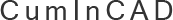CumInCAD is a Cumulative Index about publications in Computer Aided Architectural Design supported by the sibling associations ACADIA, CAADRIA, eCAADe, SIGraDi, ASCAAD and CAAD futures
 PDF papersReferences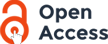id ga9917 Maia Jr., A., Valle, R. do, Manzolli, J. and Pereira, L.N.S. 1999 Generative Polymodal Music Process International Conference on Generative Art We present underlying ideas used to develop an Algorithmic Composition software named KYKLOS. It was designed to generate music based on generalised musical scales and modes. It is an interactive sonic device to be applied in composition as well in performance. The sonic output of the whole process can be described as generalised polymodal music since synthetic scales are generated by the algorithm. This environment can be used in a Computer Assisted Composition manner in order to generate MIDI files. On the other hand, it can equally be used as a performance environment in which a dynamic change of parameters enables a real time control of the sonic process. Recently, we advocated that several mathematical applications in Computer Music can be understood as Sound Functors . In extension, we describe here a sound functor used to model scales and modes. As can be verified, part of early investigations on mathematical structures in music studied musical scales and modes using Combinatorics, Fibonacci Series and Golden Mean in order to understand compositional processes which use modal concepts. Using the Functor definition it is possible to enumerate n-scales as a sequence of integers. Each value in that sequence gives the distance (in half tones) between two consecutive tones. For example, the sequence 3:2:2:3 is interpreted as a pentatonic scale C-Eb-F-G-Bb, and as defined above it is a C mode. So, if we apply cyclical permutations, (n-1)-sequences of numbers should be interpreted as n-modes of tones. Our algorithmic implementation is described briefly. A n-mode is defined as an array with n-1 integers [a1, a2, ...an-1]. Each array generated at k-th step can be read as a number a1a2a3 ....an-1 in decimal representation, where ai is a integer between 1 and 9. We denote the number obtained at k-th step as (a1a2a3 ...an-1)(k) . The rules to implement the algorithm are the following:1) V0 = (1, 1, 1, 1 ......,1) (initial n-mode)2) ? ai ? 11 with i= 1, 2...n-1 (octave range constraint)3) Vk = (a1a2a3 ...an-1)(k) < (b1b2b3 ...bn-1)(k+1) = Vk+1 where aj ?bj , 1? j ? n-1.4) Vmax = (13  n, 1, 1, ...,1)In this paper we start with a theoretical view and an introduction on the algorithmic mechanism used. Further, we present a concept of man ? machine interaction used to create the composition environment. We also describe the compositional graphic interface developed and general functions of the system. Finally there is a set of music examples generated by KYKLOS as MIDI files. other http://www.generativeart.com/ Content-type: text/plain 2003/08/07 15:25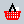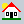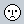CUMINCAD Papers